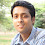### XOR (Exclusive OR) for branchless coding

The following example shows the array reversing using the XOR operator. No need to take any additional variable to reverse the array.
```int main(int argc, _TCHAR* argv[])
{
char str[] = "I AM STUDENT";
int length = strlen(str);
for(int i = 0; i < ((length/2)); i++)
{
str[i] ^= str[length - (1+i)];
str[length - (1+i)] ^= str[i];
str[i] ^= str[length - (1+i)];
}
cout << str << endl;
return 0;
}
```

The above example is one of the uses of XOR but XOR comes in handy when we can do branchless coding methods like butterfly switch etc. Sometimes this is very effective in speeding up the execution. Let's see one of the uses of XOR in branchless coding. I am taking a simple example of Y = | X |. Yes, I am generating abs of a supplied number. So, my function signature/definition in C++ looks like below:

`int absoluteBranch(int x) {    if (x < 0)     {        return -x;    }    else     {        return x;    }}`
From the C++ code, we can see the branching in code and until runtime, we can't
definitely say which branch could be executed. If we look at the assembly generated
by the code also shows branching (Without optimization):
`absoluteBranch(int):        push    rbp        mov     rbp, rsp        mov     DWORD PTR [rbp-4], edi        cmp     DWORD PTR [rbp-4], 0        jns     .L4        mov     eax, DWORD PTR [rbp-4]        neg     eax        jmp     .L5.L4:        mov     eax, DWORD PTR [rbp-4].L5:        pop     rbp        retWe have instructions like cmp(compare), jns(jump if not signed) and jmp (unconditional jump). With the help of XOR we can completely remove this branching of code while calculating abosolute of a signed number.`
`int absoluteBranchless(int x){    int y = x >> (sizeof(int) * 8 - 1);    return (x ^ y) - y;}Now I don't have any branch in the C++ code and assembly generated out ofit also branchless. Here goes the assembly (Without optimization):absoluteBranchless(int):        push    rbp        mov     rbp, rsp        mov     DWORD PTR [rbp-20], edi        mov     eax, DWORD PTR [rbp-20]        sar     eax, 31        mov     DWORD PTR [rbp-4], eax        mov     eax, DWORD PTR [rbp-20]        xor     eax, DWORD PTR [rbp-4]        sub     eax, DWORD PTR [rbp-4]        pop     rbp        retThe SAR instruction shifts the MSB and it became all FFs. For positive number it's all 00s.So for negative number mask became -1 (in two's complement) and for positive number it will always be 0. We then XOR the number with mask and subtract with mask, which effectively adds +1 in case of negative number and +0 for positive number.In above example variable y is the mask value.It's nice, we have no branching!`Remember you have shown this in office and we had some discussions on this as well.

If you cannot afford any extra memory for a new array then this XOR-ing is a good solution.I believe its used in hardware which cannot afford huge memory.